8th Grade Math Home > Teacher Resources > Secondary Mathematics Resource Booklet > Mathematics Reference Sheets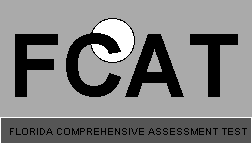Mathematics Reference Sheets

Grades 6-8 FCAT mathematics Reference Sheet

Area
KEY
 b = base h = height l = length w = width S.A. = Surface area d= diameter r = radius A = area C = circumference V = volume
Use 3.14 or 22/7 for p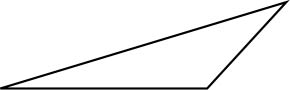Triangle

A = 1/2 bh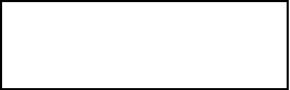Rectangle

A = lw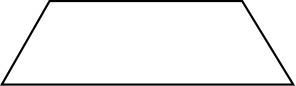Trapezoid

A = 1/2 h(b1 + b2)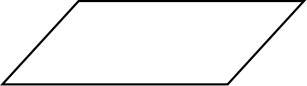Parallelogram

A = bh

Circumference
C = pd = 2pr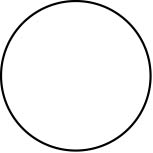Circle A = pr2

 In a polygon, the sum of the measures of the interior angles is equal to 180(n - 2), where n represents the number of sides.

Pythagorean theorem: c2 = a2 + b2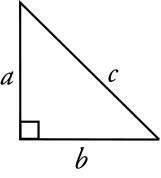Volume Total Surface Area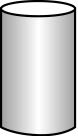Right Circular Cylinder V = pr2h S.A. = 2prh + 2pr2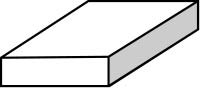Rectangular Solid V = lwh S.A. = 2(lw) + 2(hw) + 2(lh)

 Conversions 1 yard = 3 feet = 36 inches 1 mile = 1,760 yards = 5,280 feet 1 acre = 43,560 square feet 1 hour = 60 minutes 1 minute = 60 seconds 1 cup = 8 fluid ounces 1 pint = 2 cups 1 quart = 2 pints 1 gallon = 4 quarts 1 liter = 1000 milliliters = 1000 cubic centimeters 1 meter = 100 centimeters = 1000 millimeters 1 kilometer = 1000 meters 1 gram = 1000 milligrams 1 kilogram = 1000 grams 1 pound = 16 ounces 1 ton = 2,000 pounds Metric numbers with four digits are represented without a comma (e.g., 9960 kilometers). For metric number greater than four digits, a space is used instead of a comma (e.g., 12 500 liters).

Grades 9-10 FCAT mathematics Reference Sheet

Area
KEY
 b = base h = height l = length w = width= slant height S.A. = Surface area d= diameter r = radius A = area C = circumference V = volume
Use 3.14 or 22/7 for pTriangle

A = 1/2 bhRectangle

A = lwTrapezoid

A = 1/2 h(b1 + b2)Parallelogram

A = bh

Circumference
C = pd = 2prCircle A = pr2

 Volume Total Surface Area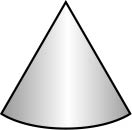Right Circular Cone V = 1/3 pr2h S.A. = 1/2 (2pr)+ pr2 = pr+ pr2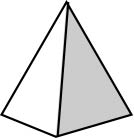Square Pyramid V = 1/3 lwh S.A. = 4(1/2 l) + l2 = 2l+ l2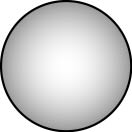Sphere V = 4/3 pr3 S.A. = 4pr2Right Circular Cylinder V = pr2h S.A. = 2prh + 2pr2Rectangular Solid V = lwh S.A. = 2(lw) + 2(hw) + 2(lh)

 In the following formulas, n represents the number of sides. In a polygon, the sum of the measures of the interior angles is equal to 180(n - 2). In a regular polygon, the measure of an interior angle is equal to [180(n - 2)] / n.

 Pythagorean theorem: c2 = a2 + b2Distance between two points P1(x1,y1) and P2 (x2,y2):y = mx + b Slope-intercept form of an equation of a line, where m = slope and b = the y-intercept: Midpoint between two points P1(x1,y1) and P2 (x2,y2):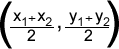d = rt Distance, rate, time formula, where d = distance, r = rate, t = time. I = prt Simple interest formula, where p = principal, r = rate, t = time.

 Conversions 1 yard = 3 feet = 36 inches 1 mile = 1,760 yards = 5,280 feet 1 acre = 43,560 square feet 1 hour = 60 minutes 1 minute = 60 seconds 1 cup = 8 fluid ounces 1 pint = 2 cups 1 quart = 2 pints 1 gallon = 4 quarts 1 liter = 1000 milliliters = 1000 cubic centimeters 1 meter = 100 centimeters = 1000 millimeters 1 kilometer = 1000 meters 1 gram = 1000 milligrams 1 kilogram = 1000 grams 1 pound = 16 ounces 1 ton = 2,000 pounds Metric numbers with four digits are represented without a comma (e.g., 9960 kilometers). For metric number greater than four digits, a space is used instead of a comma (e.g., 12 500 liters).

 FCAT MATH REFERENCE SHEET Name ______________________

DIRECTIONS: Refer to your FCAT Mathematics Reference Sheet to answer each of the following.

1. Make a drawing of a trapezoid.

2. Write the formula for the volume of a sphere. ______________
What is the volume of a sphere with a radius of 3 inches? _________________

3. Write the formula for the total surface area _____________________ and the volume ________________________ of a right circular cylinder. Use the correct formula to find how many ounces of broth could be held in a cylinder that has a radius of 2 inches and a height of 7 inches if every cubic inch of volume contains one-fourth ounce of broth. Round your answer to the nearest whole number.)

4. Find the circumference of a circle with a radius of 14 cm. Use the fractional value for pi (p) that is given on your reference sheet.

5. What is the sum of the interior angles of a regular pentagon? (5 sides)

6. If the equation of a given line is y =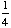x + 2, what is the slope of the line, and what is its
y-intercept? Slope _____ y-intercept _____

7. Write out the Pythagorean Theorem . _______________ The hypotenuse is represented by the letter ____ and the two legs are represented by the letters ____ and ____ . Find the length of the hypotenuse of an isosceles right triangle with legs of 5 units. Round your answer to the nearest hundredth or give the exact square root answer. _________

8. Write the formula for the distance between two points. _____________________
Use this formula to find the distance between the points (-1, -1) and (3, 3). ________

9. Write the formula for simple interest. _________________
Find the simple interest on a loan of \$300 for 8 months at 6% annual interest.

10. Write the distance, rate, time formula. ________________
Using this formula, how long does it take you to drive 780 miles at 65 mph? ______

11. Write the formula for the midpoint between two points. ___________________
Find the coordinates of the midpoint between the points (8, -3) and (-2. 11). ______

12. How many inches are in a yard? _____ How many inyard? _____

13. There are _____ feet in a mile. What part of a mile is 1320 feet? _____

14. How many cups are in one gallon? _________

15. How many ounces are in one quart? _________

Find the area of each figure.

 16.17.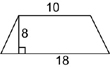18.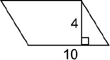Find the volume of each figure.

 19.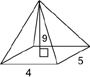20.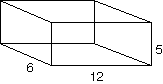Used by permission of Cheryl Greenfield, Gibbs high School, 2002.

Copyright Statement for this Assessment and Evaluation Services Publication

Authorization for reproduction of this document is hereby granted to persons acting in an official capacity within the State System of Public Education as defined in Section 228.041(1), Florida Statutes. The copyright notice at the bottom of this page must be included in all copies.